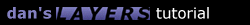2.2 Parabola

Parabolas can be used to simulate objects falling under gravity. When using the parabola function below, you first have to figure out how many pixels you want to move the layer in both the horizontal and the vertical (these are absolute values - no negative values needed). You can define any of 4 directions to move the layer (up, down, left, right) by changing the xdirection and the ydirection. Using these values the function plots out the location along a parabolic curve.

I have incorporated 2 different types of parabolas in this function - you change them by setting the variable "ParType" to 1 or 2.

ParType 1 is what I call a dropping parabola. It starts at the vertex, and moves outward from there - similar to how an object would be dropped out of a plane.

ParType 2 is what I call an arcing parabola. It starts at the bottom (if you set the direction to -1) and climbs along a parabolic arc and then comes back down - similar to how a baseball travels when you hit it in the air.

```function parabola() {
ParLayername = "layer1";   // name of the layer you want to move
ParType = 1;               // the type of parabola you want to make
// ParType = 1 means a dropping parabola
// ParType = 2 means an arcing parabola
ParXdistance = 200;        // total horizontal distance to move
ParYdistance = 200;        // total vertical distance to move
ParXdirection = 1;         // use 1 to move right, -1 to move left
ParYdirection = 1;         // use 1 to move down, -1 to move up
ParHorizontal = 2;         // horizontal incrementation
ParSpeed = 20;             // speed of repetition
ParI = 0;
ParLeft = document.layers[ParLayername].left;
ParTop = document.layers[ParLayername].top;
ParMultiplier = ParYdistance/Math.pow(ParXdistance/ParType,2);
slideparabola();
}

function slideparabola() {
if (Math.abs(ParI) < Math.abs(ParXdistance)) {
ParI += ParXdirection*ParHorizontal;
var x = ParLeft + ParI;
if (ParType == 1)
var y = ParTop + ParYdirection*ParMultiplier*Math.pow(Math.abs(ParI),2);
if (ParType == 2)
var y = ParTop + ParYdirection*ParYdistance - ParYdirection*ParMultiplier*Math.pow(ParXdistance/2-Math.abs(ParI),2);
document.layers[ParLayername].moveTo(x,y);
setTimeout("slideparabola()", ParSpeed);
}
}
```

Now in the type 2 example, I wanted the movement to end when the layer got back to the same vertical position - the top value is the same when it starts and ends. It may be useful to have the movement end before or after it reaches that same vertical postion. For example, say you wanted to simulate something being thrown off of a cliff - you'd throw it up and then it would come back down to the same level and keep falling some distance further. If you wanted to do that you'd have to change the "if" statement in the "parabola()" function.

If you wanted the layer to fall to a vertical position of 400 you would replace this line:

```	if (Math.abs(ParI) < Math.abs(ParXdistance)) {
```

With this line:

```	if (document.layers[ParLayername].top < 400) {
```

You could also stop the movement using horizontal values like:

```	if (document.layers[ParLayername].left < 250) {
```1.1 Introduction 1.2 Overlapping 1.3 Nesting 1.4 Using JavaScript 2.1 Sliding Layers 2.2 Pre-Built Functions 2.3 Clipping Layers 2.4 Looping Animations 2.5 Changing Images 3.1 Mouse-Click Animation 3.2 Capturing Keystrokes 3.3 Drag and Drop 4.1 Making Demos 4.4 Problems 4.5 Screen Sizes Copyright © 1997 Dan Steinman. All rights reserved.